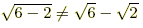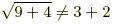# square root rules

Square Root Rules

Algebra rules for square roots are listed below. Square root rules are a subset of nth root rules and exponent rules.

 Definitions1.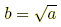if both b ≥ 0 and b2 = a.2.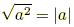Examples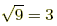because 32 = 9.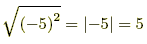3. If a ≥ 0 then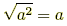.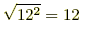Distributing (a ≥ 0 and b ≥ 0)1.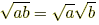2.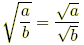(b ≠ 0)3.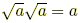Examples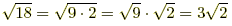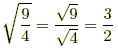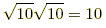4.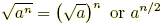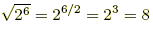Rationalizing the Denominator(a > 0, b > 0, c > 0) Examples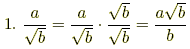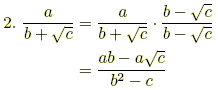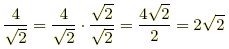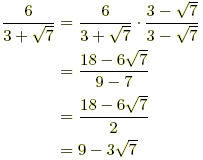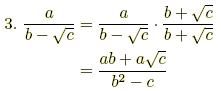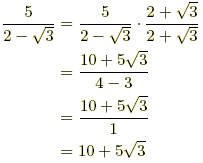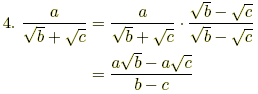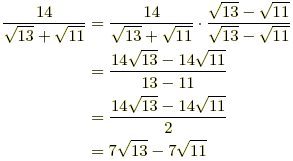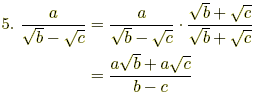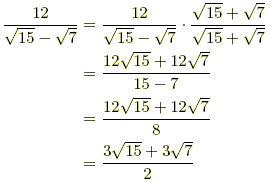Careful!!1.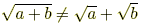2.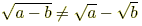3.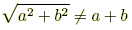Examples